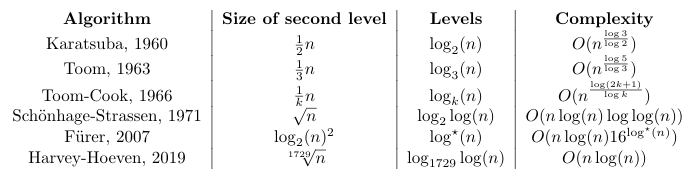# Complexity of integer multiplication almost solved

Whilst not quite as close as the proofs of the ternary Goldbach conjecture and bounded gaps between primes, there has been a quick succession of two important and somewhat complementary breakthroughs on the computational complexity of integer multiplication:

• Afshani, Freksen, Kamma, and Larsen proved a lower bound of Ω(n log n) on the circuit complexity of integer multiplication, conditional on a conjecture in network coding.
• Harvey and van der Hoeven published an algorithm for large integer multiplication, establishing an unconditional upper bound of O(n log n). This is only marginally faster than the O(n log n log log n) Schönhage–Strassen algorithm, overtaking it only for unimaginably large numbers, but is of great theoretical interest because it coincides with the conjectural lower bound. (The authors also showed that the same complexity can be achieved by a multi-tape Turing machine.)

Essentially all modern integer multiplication algorithms are recursive in nature, and the computational complexity depends on the number of levels of recursion together with computational complexity of each level. To summarise:In practice, it is common to mix-and-match these algorithms: using FFT-based algorithms (typically Schönhage–Strassen) near the root of the recursion, and switching to Toom-Cook at lower levels, before finally falling back on hardware multiplication at the leaves. This new Harvey–Hoeven algorithm is only suitable for really large integers, and switches to older algorithms (in the manner described) for numbers with fewer than 2^(1729^12) binary digits.

A refinement of the algorithm reduces that to 2^(9^12) = 2^282429536481 binary digits, but that is still much much larger than any number that could be practically stored, even storing one digit per atom in the observable universe.

This entry was posted in Uncategorized. Bookmark the permalink.